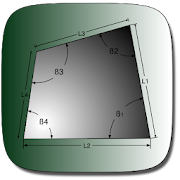# Geometry, Area and Perimeter CalculatorEveryone
18
With the Geometry Calculator, learn "How to" calculate area, perimeter, angles, sides, hights, ... of a lot of geometric figures.

A1) Calculate Equilateral Triangle: Calculate Area of an Equilateral Triangle using only one variable. Calculate the height, the perimeter and the Area of any Equilateral Triangle.
A2) Right Triangle. Calculate the area of a right Triangle, the perimeter or the height. Calculate easily the not right angle of a the right triangle
A3) Isosceles Triangle. Calculate the area of a Isosceles Triangle, Discover how to calculate the rest of the values using only two of them. Calculate area, perimeter, sides, height and angle quickly and easily
A4) Scalene Triangle. Calculate all variable of a Scale Triangle. Calculate area, perimeter, the three sides and the three angles.

Calculate more geometric figures:

- Square
- Rectangle
- Rhombus
- Rhomboid

- Right Trapezoid
- Isosceles Trapezoid

- Pentagons
- Hexagons
- Heptagons
- Octagons

.... easy.... fast.....
Collapse

Review Policy
4.1
18 total
5
4
3
2
1

## What's New

Some translation corrections
Collapse

Updated
November 19, 2018
Size
7.2M
Installs
5,000+
Current Version
1.1.2
Requires Android
4.4 and up
Content Rating
Everyone
Permissions
Offered By
AMI DIGITAL EDITIONS, SL